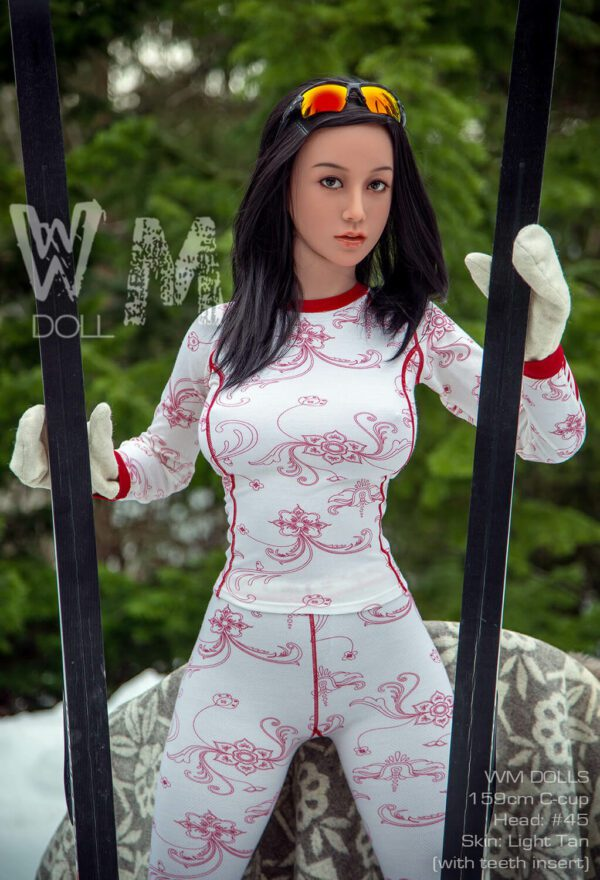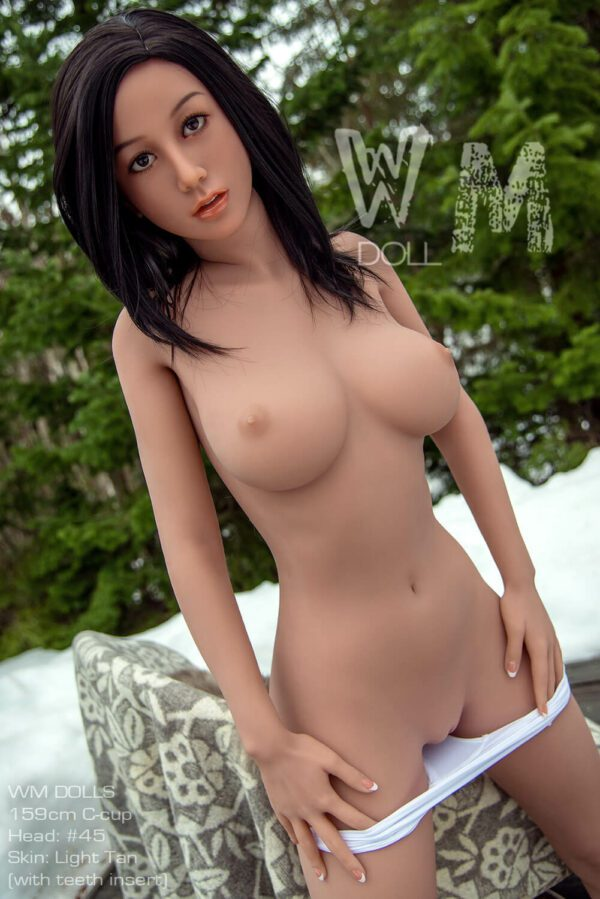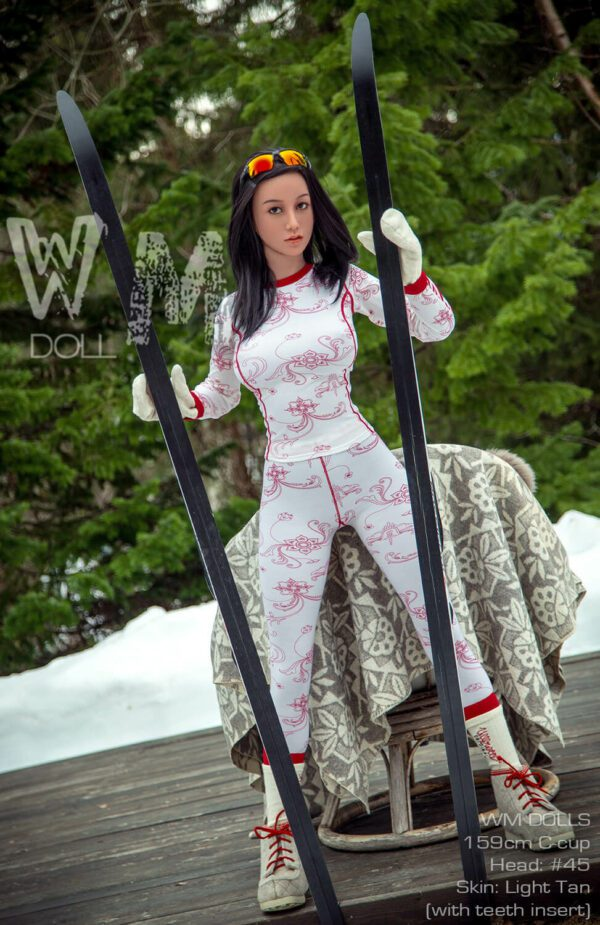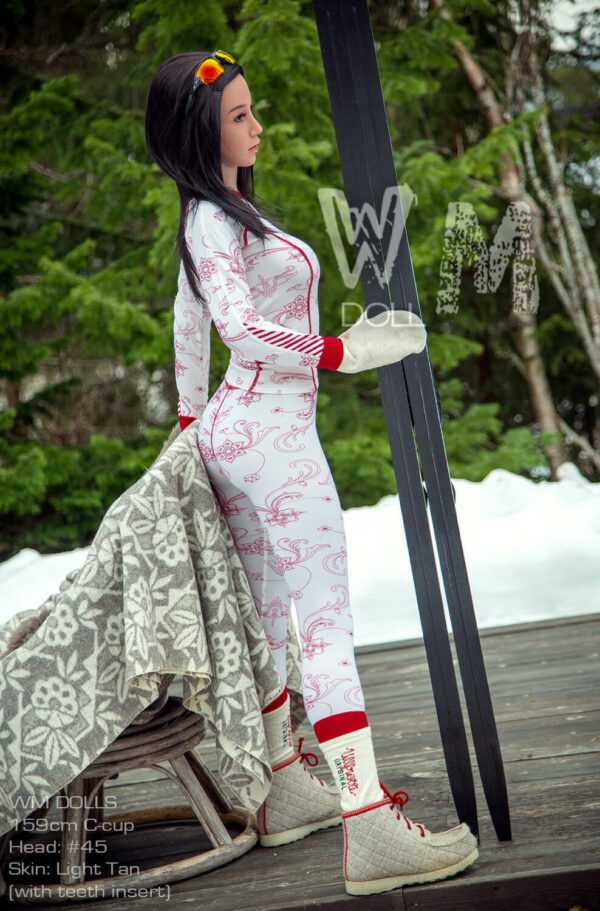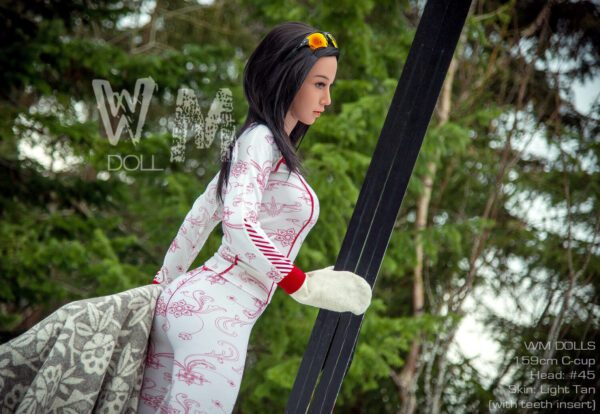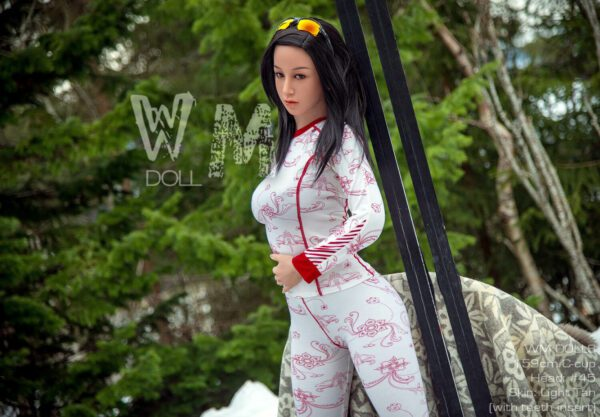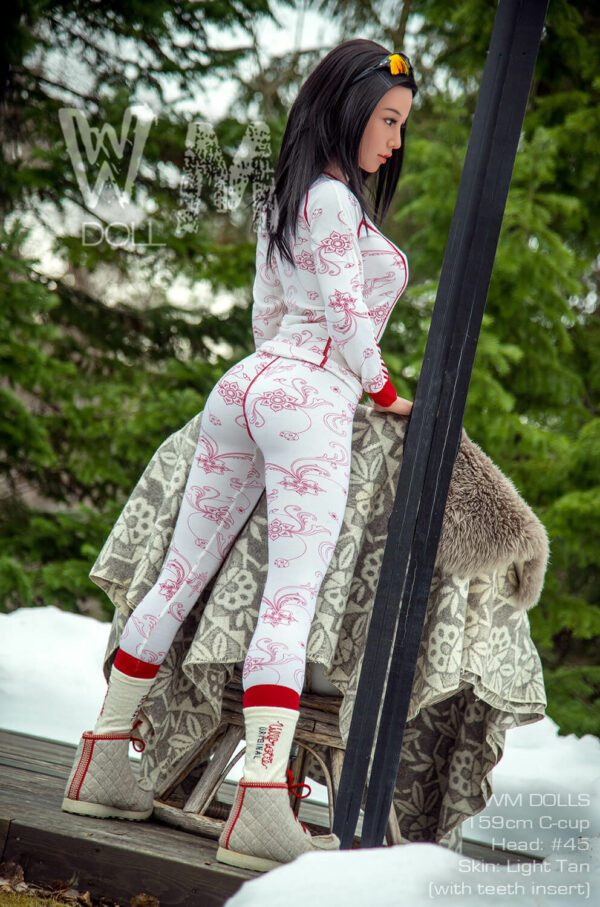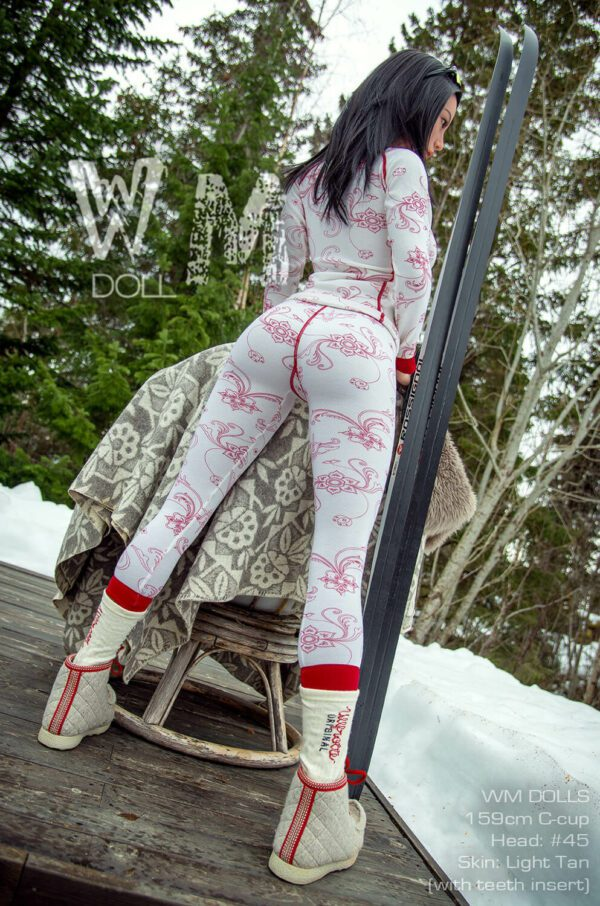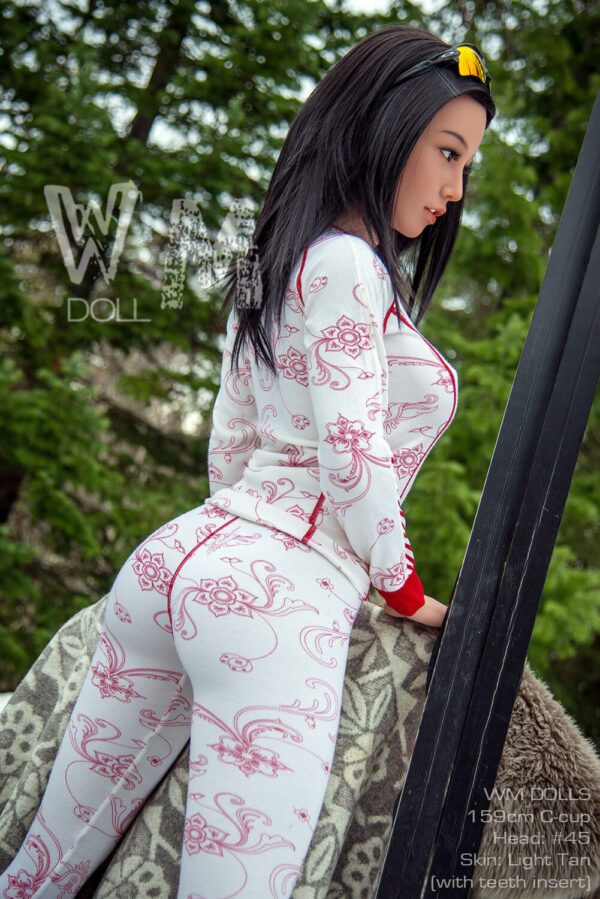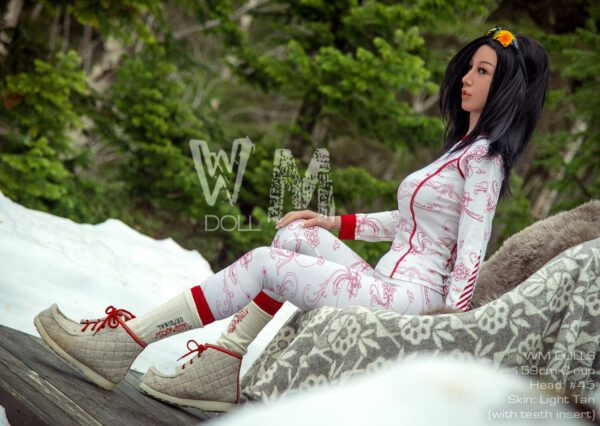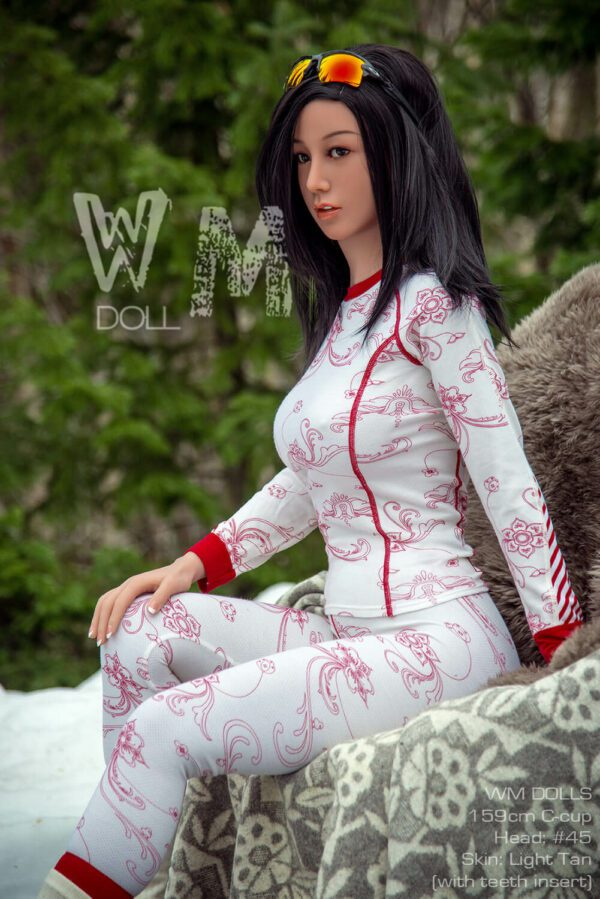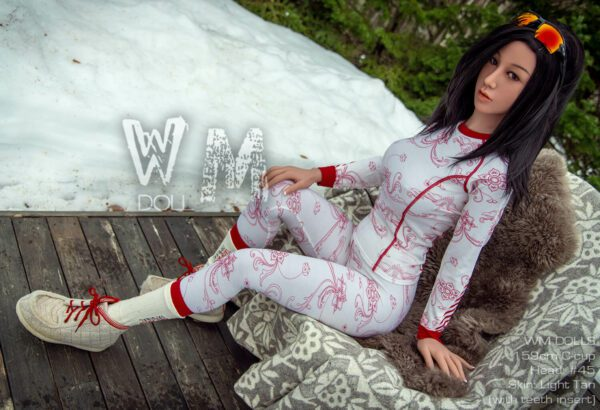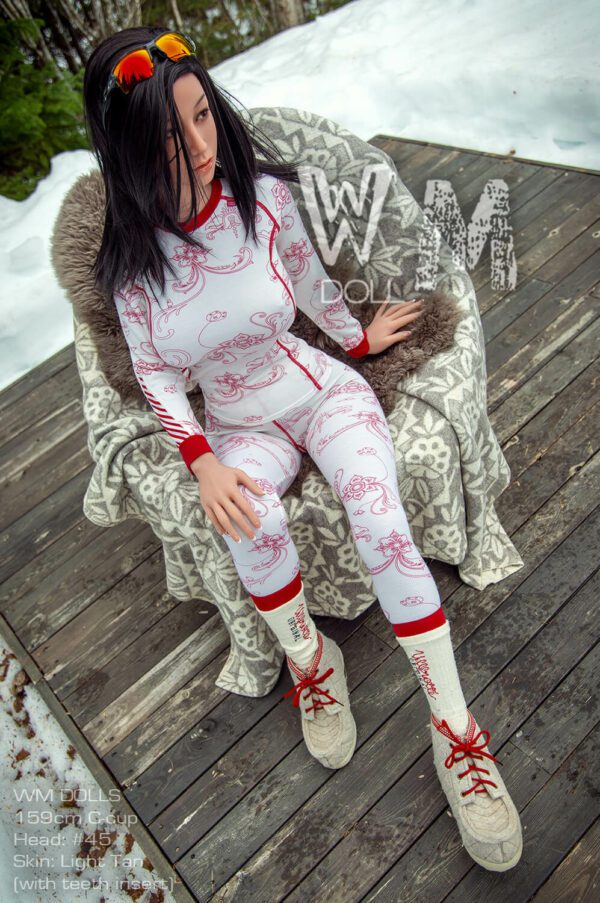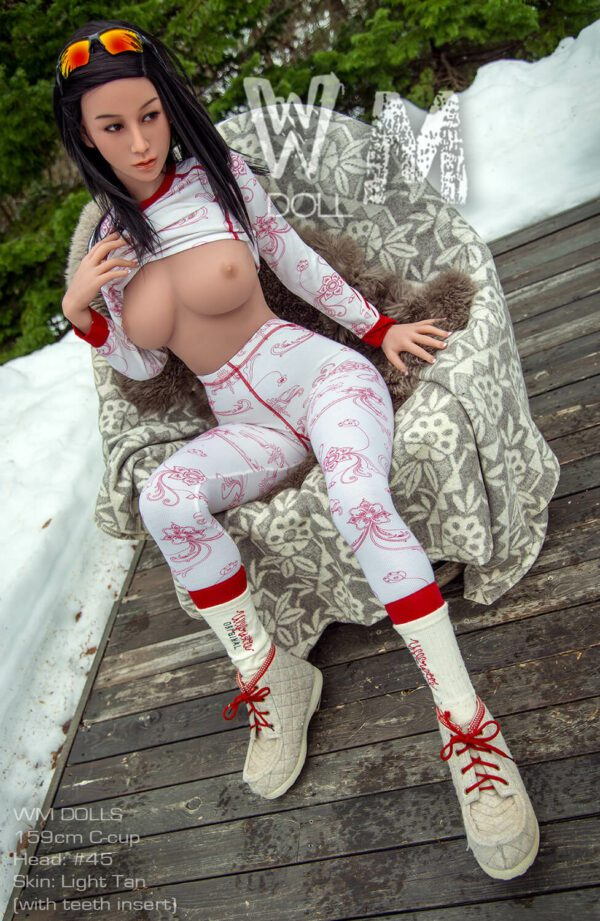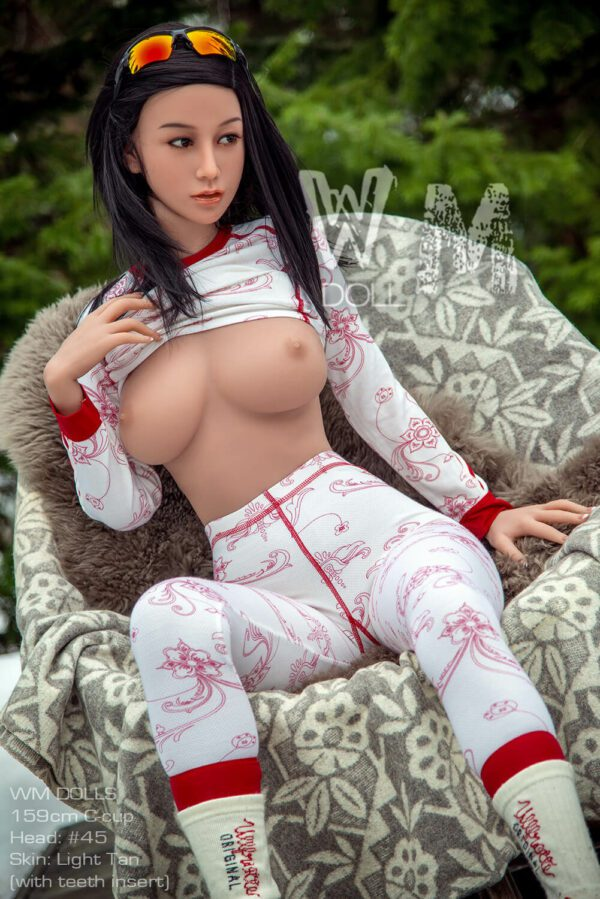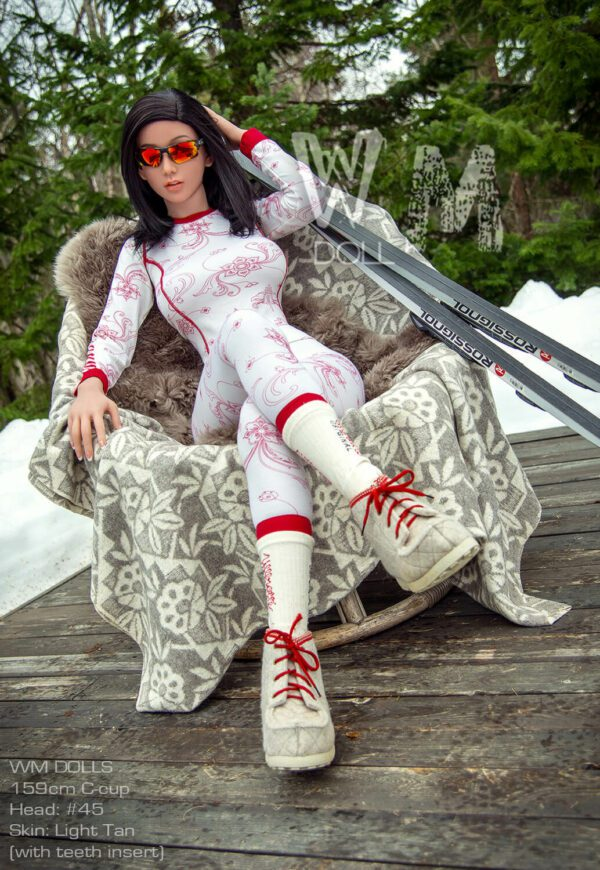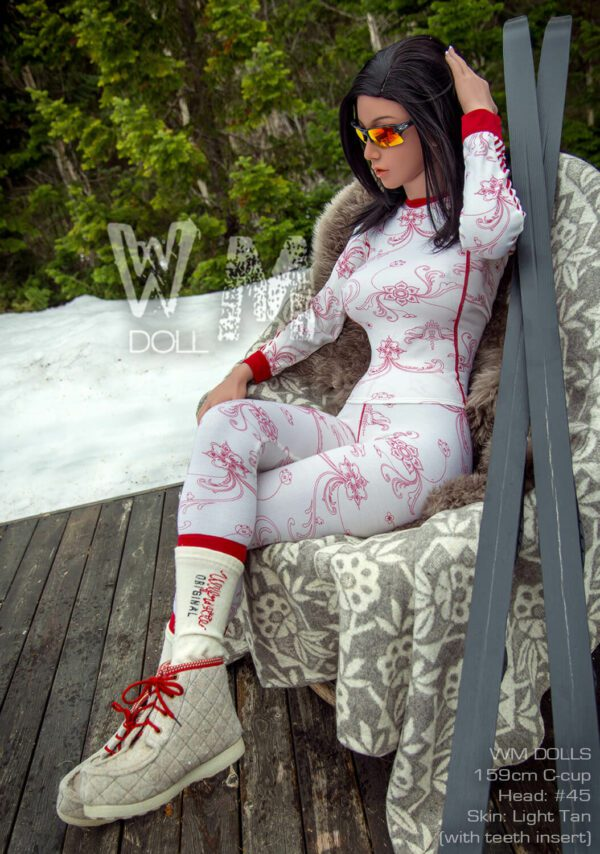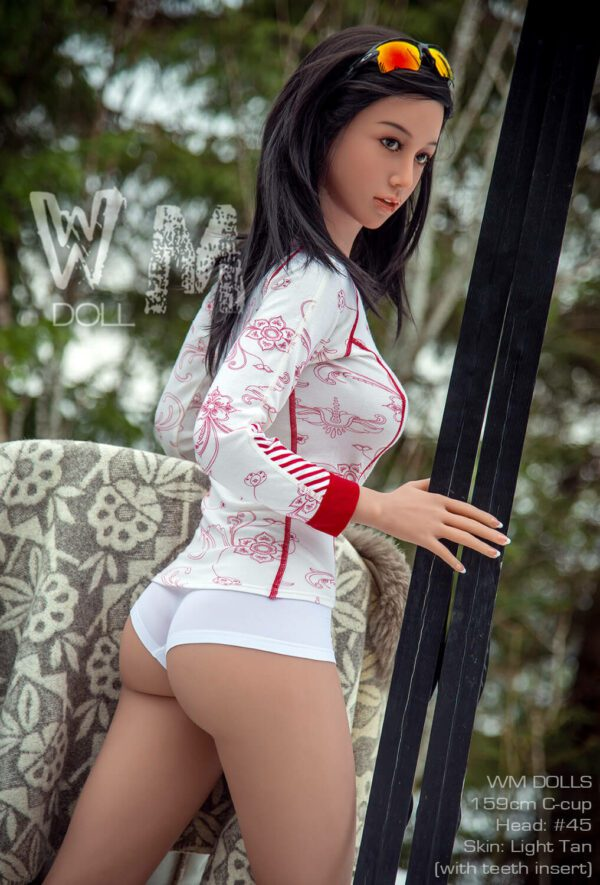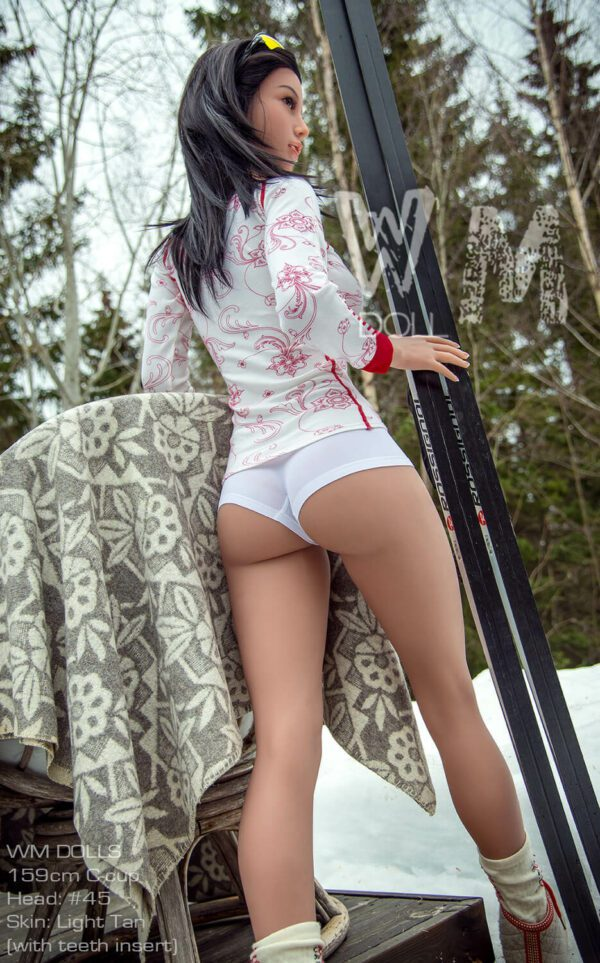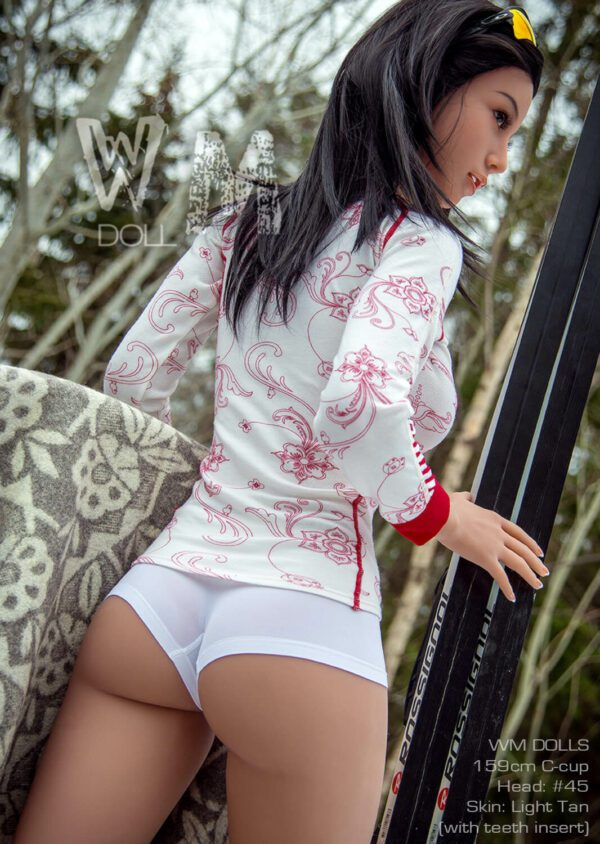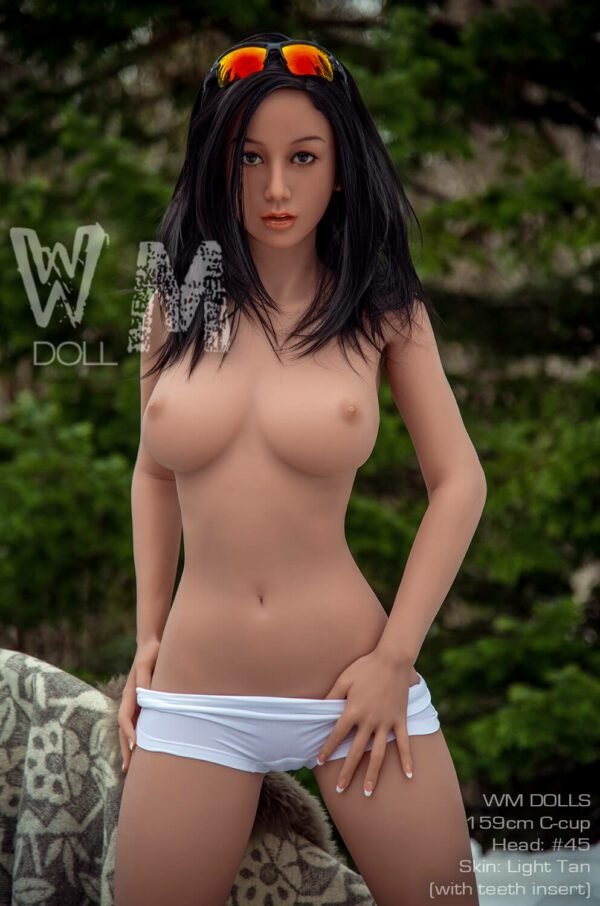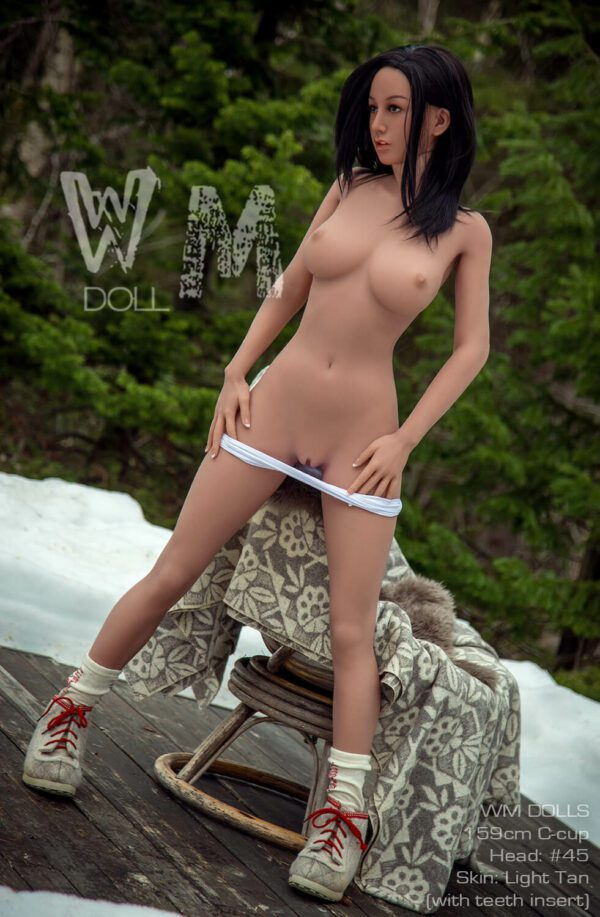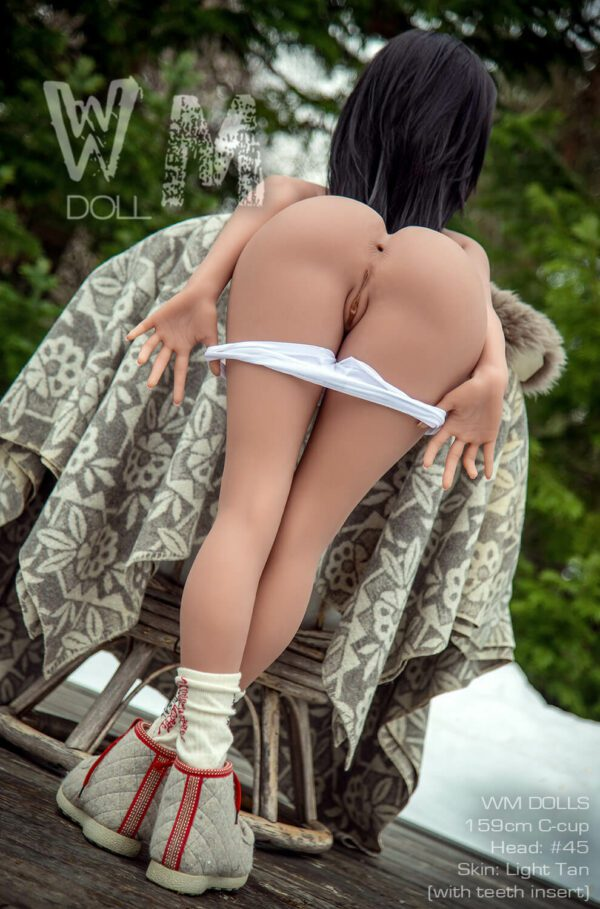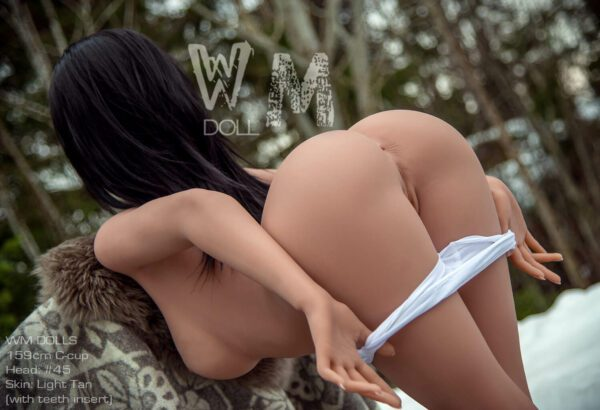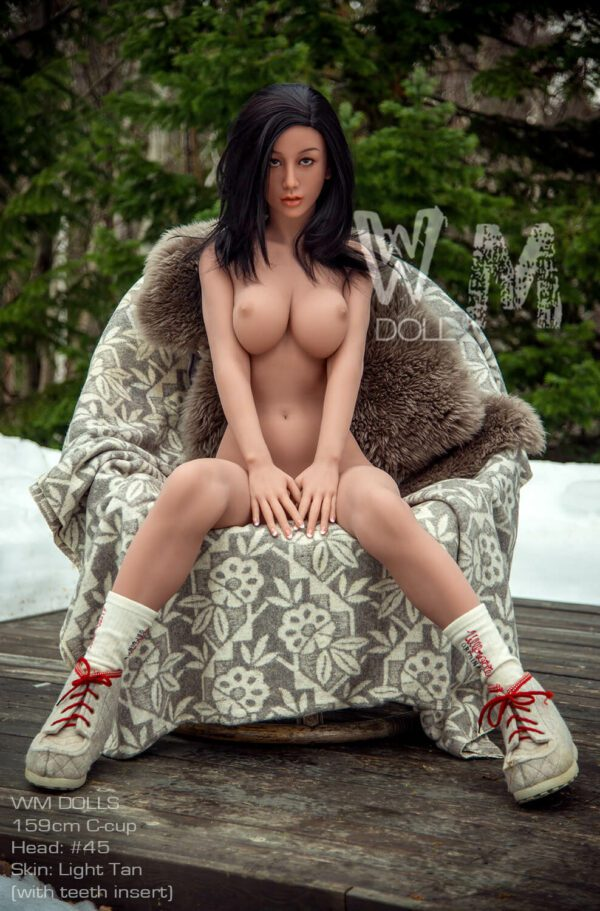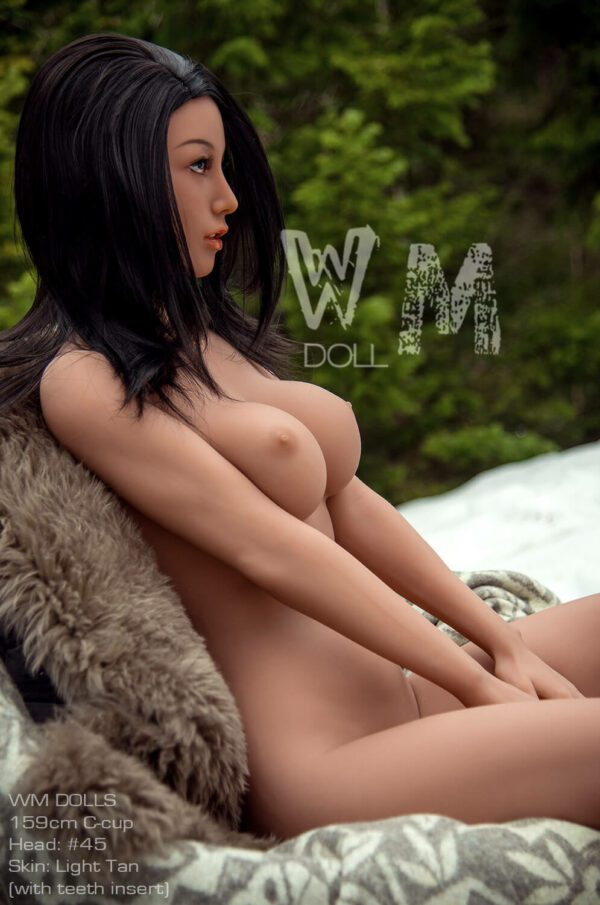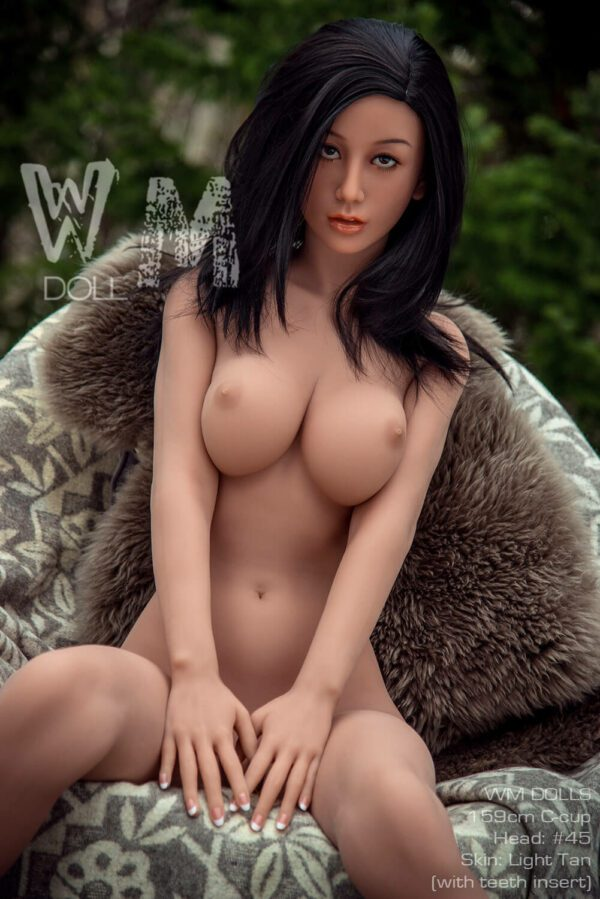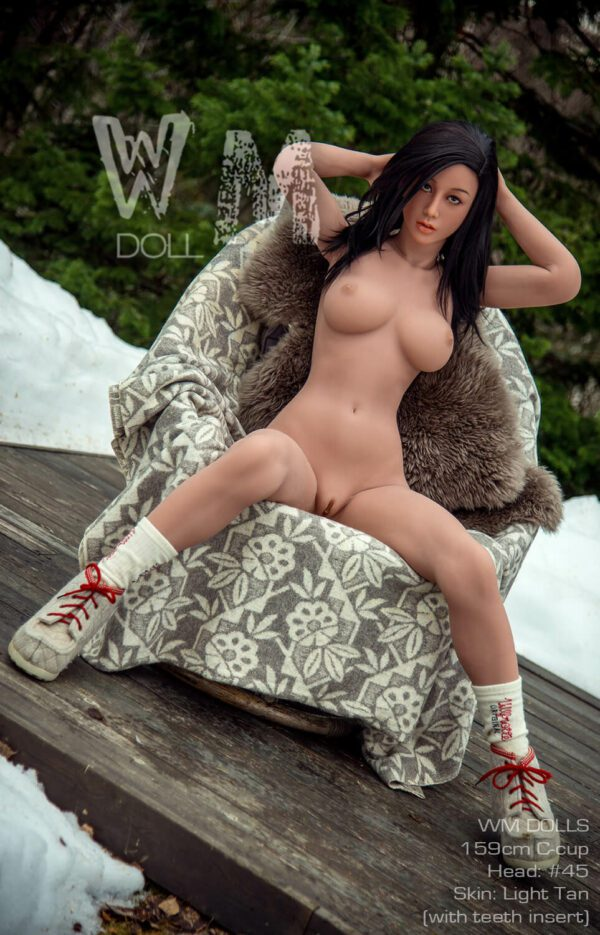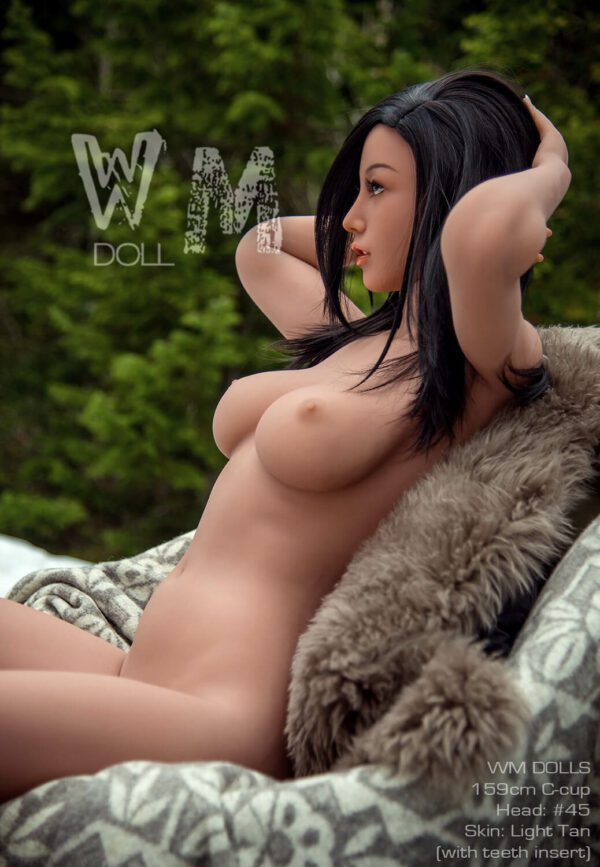# Anastasia – 5’3″ | 159cm Hot Sex Doll

WM Doll
\$1,800

Anastasia – 5’3″ | 159cm Hot Sex Doll made with high quality and safety standards by WM Doll, this genuine silicone and TPE sexy real sex doll is CE certified. With various features complementing each other, this realistic sex doll is sure to please the owner.

## Description

Height > 5ft 1.5in – 5ft 3in (156cm – 160cm), Ethnicity > American, Best Sellers, Hair Color > Black, Body Style > Curvy, Material > Fully TPE, Ethnicity > Interracial, Ethnicity > Latina, Breast Size > Medium, Body Style > Petite, See All Sex Dolls, BRANDS > WM Doll, Age > Young Adult

• \$
• \$
• \$
• \$
• \$
• \$
• \$
• \$
• \$
• \$
• \$
• \$
• \$
• \$
• \$
• \$
• \$
• \$
• \$
• \$
• \$
• \$
• \$
• \$
• \$
• \$
• \$
• \$
• \$
• \$
• \$
• \$
• \$
• \$
• \$
• \$
• \$
• \$
• \$
• \$
• \$
• \$
• \$
• \$
• \$
• \$
• \$
• \$
• \$
• \$
• \$
• \$
• \$
• \$
• \$
• \$
• \$
• \$
• \$
• \$
• \$
• \$
• \$
• \$
• \$
• \$
• \$
• \$
• \$
• \$
• \$
• \$
• \$
• \$
• \$
• \$
• \$
• \$
• \$
• \$
• \$
• \$
• \$
• \$
• \$
• \$
• \$
• \$
• \$
• \$
• \$
• \$
• \$
• \$
• \$
• \$
• \$
• \$
• \$
• \$
• \$
• \$
• \$
• \$
• \$
• \$
• \$
• \$
• \$
• \$
• \$
• \$
• \$
• \$
• \$
• \$
• \$
• \$
• \$
• \$
• \$
• \$
• \$
• \$
• \$
• \$
• \$
• \$
• \$
• \$
• \$
• \$
• \$
• \$
• \$
• \$
• \$

• \$
• \$
• \$
• \$
• \$
• \$
• \$

• \$

• \$
• \$
• \$
• \$
• \$
• \$
• \$
• \$
• \$
• \$
• \$
• \$
• \$
• \$
• \$
• \$
• \$
• \$
• \$
• \$
• \$

• \$
• \$
• \$
• \$
• \$
• \$
• \$
• \$
• \$
• \$
• \$
• \$
• \$
• \$
• \$
• \$
• \$
• \$
• \$
• \$

• \$
• \$
• \$
• \$
• \$
• \$
• \$

• \$
• 40 \$

• \$
• 100 \$

• \$
• \$
• \$
• \$
• \$

• \$
• \$
• \$
• \$
• \$

• \$
• \$
• \$

• \$
• 130 \$

• \$
• \$
• \$

• \$
• 40 \$

• \$
• \$
• \$
• \$

• \$
• \$
• \$
• \$
• \$

• \$
• \$

• \$
• \$
• \$
• \$
• \$
• \$
• \$
• \$
• \$
• \$

• \$
• \$
• \$
• \$
• \$
• \$

• \$
• 200 \$

• \$
• 100 \$

• \$
• 675 \$

• \$
• 390 \$

## Doll Measurements

Height:159cm | 5 ft 2.5 in
Weight: 36kg | 79.3 lbs
Full Bust: 83 cm | 32.6 in
Under Bust 69 cm | 27.1 in
Cup Size : C
Waist: 57 cm | 22.4 in
Hips: 90 cm | 35.4 in
Shoulder: 34 cm | 13.3 in
Vaginal Depth: 18cm | 7 in
Anal Depth: 15 cm | 5.9 in
Oral Depth: 12 cm | 4.7 cm